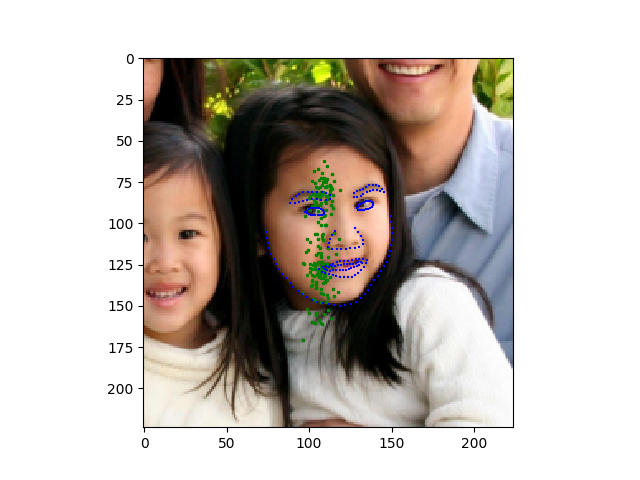# Any help for a facial keypoint detection model?

Hi !

I’m training a model for facial keypoints detection, using the Helen dataset.
I’m using a Imagenet pretrained mobilenetV2 as backbone, retraining only the final layers for 10 epochs on the full dataset.
I’m getting very poor results and I wanted to know whether someone could help me out. Basically, the model sticks all the points in the center and after the first epoch both the training and validation losses stop decreasing.I’m not doing particularly fancy things and I don’t see what prevents training.
First, for the model, :

``````backbone =  models.mobilenet_v2(pretrained = False)

features = backbone.features
Flatten(),
nn.Linear(1280, 1000),
nn.Tanh(),
nn.Linear(1000, 1000),
nn.Tanh(),
nn.Linear(1000,512),
nn.ReLU(inplace = True),
nn.Linear(512,
for p in features.parameters():
self.predictor = nn.Sequential(features, predictions)

``````

Then, for training, I do:

``````predictor = FaceModel()
loss_fn = nn.MSELoss(size_average = False)

for epoch in range(epochs):
predictor = predictor.to(device)
predictor.train()
train_loss = 0.

x = sample['image']
y = sample['points']

preds = predictor(x.to(device))
loss = loss_fn(preds, y.to(device))

loss.backward()